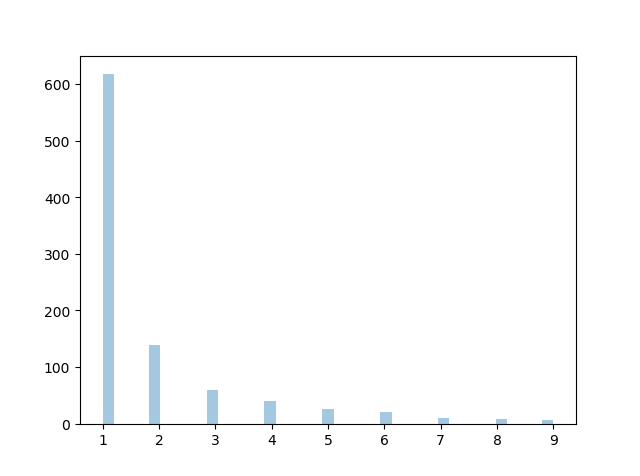THE WORLD'S LARGEST WEB DEVELOPER SITE

# Zipf Distribution

Zipf distritutions are used to sample data based on zipf's law.

Zipf's Law: In a collection the nth common term is 1/n times of the most common term. E.g. 5th common word in english has occurs nearly 1/5th times as of the most used word.

It has two parameters:

`a` - distribution parameter.

`size` - The shape of the returned array.

### Example

Draw out a sample for zipf distribution with distribution parameter 2 with size 2x3:

from numpy import random

x = random.zipf(a=2, size=(2, 3))

print(x)
Try it Yourself »

## Visualization of Zipf Distribution

Sample 1000 points but plotting only ones with value < 10 for more meaningful chart.

### Example

from numpy import random
import matplotlib.pyplot as plt
import seaborn as sns

x = random.zipf(a=2, size=1000)
sns.distplot(x[x<10], kde=False)

plt.show()

### ResultTry it Yourself »